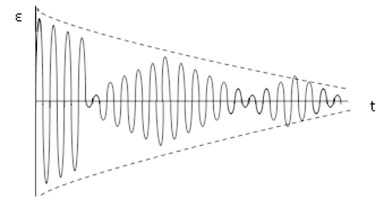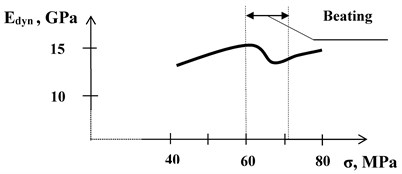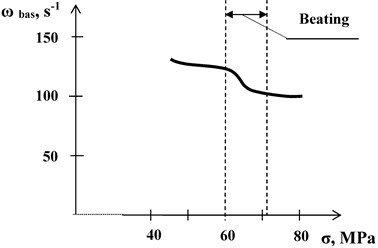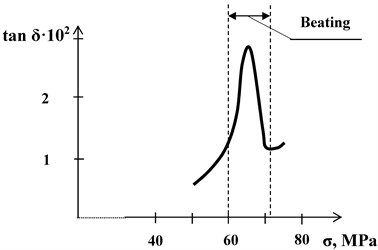Published: 07 October 2016

# Nonlinear dynamic effect in synthetic fibres from semi- and rigid chain polymers

Alla A. Romanova1
Pavel P. Rymkevich2
1, 2Military Space Academy by A. F. Mozhayskii, St. Petersburg, Russia
Corresponding Author:
Pavel P. Rymkevich
Views 9

#### Abstract

A study on elastic and relaxation properties of a set of oriented polymers in the dynamic strain mode is reported in this paper. It is established that all investigated objects show the beatings phenomenon at a certain range of stresses and temperatures. The model and possible mechanisms of the observed phenomena are offered.

## 1. Introduction

High tenacity synthetic fibres based on semi- and rigid chain polymers and fibrous composite materials are widely used in the technical fields of application, particularly, in aerospace engineering, parachute production and anti-vibration systems. In real-life conditions of production and operation uniaxially oriented polymers undergo a complicated mechanical effect, including not only static loading, but also periodic strain. It is the dynamic nonlinear processes where the relaxation properties of polymers are clearly seen.

## 2. Experiment

Here we have investigated the synthetic fibres formed of semi- and rigid chain polymers, such as poly-$p$-phenylene terephthal amide (PPTA, trade mark Terlon, the Russian analogue of Kevlar), poly-$p$-benzo imidazole (PBI, Russian trade mark SVM), polyethylene terephthalate (PET, Russian trade mark Lavsan).

The investigation of elastic and relaxation properties of synthetic fibres in the dynamic non-destructive strain mode was conducted using the free longitudinal oscillation method at the maximum stresses not exceeding 40-50 % of ultimate tensile strength . The temperature test was conducted in the temperature range of 20-450°C.

The choice of the range of mechanical stresses was made based on the criterion that the fibres are limited to 40-50 % of the tensile stress. During the determining of the stress in a specimen, it was suggested that there is a no-significant change in specimen section at various tensile loads, and this change may be ignored. The measurements have been taken at the load step of 5-10 N depending on the investigated material.

Before each test, all the specimens were dried in a desiccator at a relative humidity of 65 % for not less than 2 days; in order to detach a part of plastic strain and straighten the mechanical “memory” the specimens were placed under minimal test load 2 N for 30 minutes.

The test in the context of the investigation of the impact of the level of mechanical stress on dynamic characteristics was conducted isothermally at an ambient temperature of 20±2 °С and relative humidity of 65±5%. The fibre clamping length was 200 mm.

The temperature test was conducted in the temperature range of 20-450 °C. The rate of temperature increase was ~5 /min. The temperature gradient at the specimen length did not exceed 3-6 °C.

The amplitude of oscillations imparted to the specimen did not exceed 0,5 % of the clamping length.

## 3. Test results and discussion

We have determined that all the investigated fibres within a certain range of mechanical stress (or the levels of static strain) exhibit a complicated nonexponential form of decaying oscillations, or beatings. The form being hard to explain traditionally is represented schematically in Fig. 1.

Fig. 1Beatings (amplitude-modulated free oscillations)The equation for the dynamic part of the strain can be visually demonstrated as follows:

1
${\epsilon }_{dyn}\left(t\right)={C}_{0}{e}^{-{B}_{0}t}+{C}_{1}{e}^{-{B}_{1}t}\mathrm{sin}\left({\omega }_{1}t+{\phi }_{1}\right)+{C}_{2}{e}^{-{B}_{2}t}\mathrm{sin}\left({\omega }_{2}t+{\phi }_{2}\right)+\delta \epsilon \left(t\right),$

where ${C}_{i}$ are the initial amplitudes of oscillations; ${\omega }_{bas}=\left({\omega }_{1}+{\omega }_{2}\right)/2$ is the basic (beating) angular frequency of oscillations; ${\omega }_{mod}=\left({\omega }_{1}-{\omega }_{2}\right)/2$ is the angular frequency of modulation of amplitude; $\phi$ is the initial phase.

The residual $\delta \epsilon \left(t\right)$ forms the so-called “white noise”. Analysis revealed that the noise does not exceed the error range of 1-3 %.

It should be noted that the free exponent value is traditionally low, $\left|{C}_{0}\right|\le 0,1\left|{C}_{1}\right|$. The presence of the free exponent was discovered earlier in study  through the torsional oscillation method for a number of caoutchoucs and represented in a 3D model. In our opinion, the presence of the free exponent reflects a transition process. Its existence has been shown in the works of Yu. N. Rabotnov .

The main behaviour characteristics of synthetic fibres in the nonexponential decay mode should also be noted.

1) The beatings are observed in all investigated fibres.

2) The beatings exist in a wide range of temperatures up to the glass transition temperature. However, at the glass transition temperature this phenomenon disappears spasmodically within the whole load interval.

3) The beatings phenomenon exists at a certain stress interval (or the levels of static strain) appearing and disappearing spasmodically.

4) In the case of the coincidence of frequencies ${\omega }_{1}$ and ${\omega }_{2}$ an acute maximum of the tangent of the mechanical loss angle (Fig. 2(c)) takes place, which shows its resonant behaviour. Nevertheless, the elastic modulus $E\text{'}$, calculated in a traditional way  ($E\text{'}=\frac{m}{F}{\omega }_{bas}^{2}$, where $F=S/l$ is the specimen form-factor, $m$, $l$, $S$ are mass, length and area of fibres cross-section respectively) shows the minimum. (Fig. 2(a)).

5) Thus, it is possible to shift the mechanical loss maximum on the stress scale in either direction by changing the length of fibres (the base).

6) The frequency ${\omega }_{bas}$ near the beatings significantly depends on the load, while this dependency is weak outside the area of the beatings (Fig. 2(b)).

7) The frequency ${\omega }_{mod}$ does not depend on the specimen length and section.

Fig. 2Dependencies of dynamic modulus of elasticity Еdyn (a), basic frequency of oscillations ωbas(b), and tan δ of mechanical losses (c) on basic stress σ for PET fibres at T= 20 °Ca)b)c)

Applying the well-known method based on the Boltzmann-Volterra equation [7-9], the nonlinear complicated strain mode can be viewed as an interaction of static and dynamic parts of the hereditary relaxation core. In this case the following applies to the periodic processes:

2
${\sigma }_{dyn}\left(t\right)={E}_{0dyn}{\epsilon }_{dyn}\left(t\right)-\underset{0}{\overset{1}{\int }}{r}_{dyn}\left({\epsilon }_{st},t-\theta \right){\epsilon }_{dyn}\left(\theta \right)d\theta ,$

where $t$ is time; ${\epsilon }_{st}$ is level of static strain; ${\sigma }_{dyn}\left(t\right)$ is mechanical stress corresponding to periodic strain ${\epsilon }_{dyn}\left(t\right)$; ${E}_{0dyn}$ is initial dynamic modulus of elasticity; ${r}_{dyn}\left({\epsilon }_{st},t\right)$ is hereditary dynamic core of the level of static strain ${\epsilon }_{st}$. In this connection it is suggested that the dynamic loading takes place in quasiequilibrium static strain, for which the following equation applies ${\epsilon }_{st}{\tau }_{dyn}\ll 1$, where ${\tau }_{dyn}$ is the characteristic time of the dynamic process.

In order to quantitatively describe the complicated form of the observed oscillating process a simulation model may be suggested, which allows distinguishing the basic and additional oscillation frequencies and their amplitudes using the Fourier transform.

In the context of such an approach the Laplace transformation of dynamic strain is represented as follows:

3
$\stackrel{-}{\epsilon }\left(s\right)=\frac{s\epsilon \left(0\right)+\stackrel{˙}{\epsilon }\left(0\right)}{{s}^{2}+{\stackrel{-}{{\omega }_{0}}}^{2}-\stackrel{-}{{r}_{dyn}}\left(s\right)},$

where $s$ is Laplace parameter; $\epsilon \left(0\right)$ and $\stackrel{˙}{\epsilon }\left(0\right)$ are initial (random) conditions; $\stackrel{-}{{r}_{dyn}}\left(s\right)$ is image of the hereditary dynamic core; ${\stackrel{-}{{\omega }_{0}}}^{2}=\frac{{S}_{0}{E}_{0dyn}}{ml}$, here $\frac{ml}{{S}_{0}}=F$ is form-factor of the investigated specimen.

Conceptually, the image of dynamic relaxation core can be demonstrated as follows:

4
$\stackrel{-}{{r}_{dyn}}\left(s\right)=\sum _{\left(n\right)}{q}_{n}\frac{1}{1+s{\tau }_{n}}.$

Here ${q}_{n}$ is spectral intensity of the corresponding relaxation process with the relaxation time ${\tau }_{n}$.

It can be shown that the dynamic part of the core looks as follows:

5
${r}_{dyn}\left(t\right)={M}_{1}{e}^{-{\nu }_{1}t}+{e}^{-{\nu }_{2}t}\left({M}_{2}\mathrm{cos}\xi t+\frac{{M}_{3}-{M}_{2}{\nu }_{2}}{\xi }\mathrm{sin}\xi t\right),$

where ${M}_{i}\left(i=1,2,3\right)$, ${\nu }_{i}\left(i=1,2,3\right)$, $\xi$ are characteristics of the dynamic core. Overall, Eq. (5) corresponds with Yu. N. Rabotnov’s views  which support the existence of relaxation cores of an oscillation type.

The following interrelation of the tangent of the mechanical loss angle and parameters of dynamic hereditary relaxation core has been found:

6
$\mathrm{tan}\delta =\frac{\left[{M}_{2}\left({W}^{2}-{\omega }_{bas}^{2}\right)-2{\nu }_{2}{M}_{3}\right]{\omega }_{bas}^{2}}{\left[{\left({W}^{2}-{\omega }_{bas}^{2}\right)}^{2}+4{\omega }_{bas}^{2}{\nu }_{2}^{2}\right]{\stackrel{-}{{\omega }_{0}}}^{2}},$

where ${W}^{2}={\xi }^{2}+{\nu }_{2}^{2}$; $\xi$ is core oscillation frequency; ${\nu }_{2}$ is core decay rate,${\stackrel{-}{{\omega }_{0}}}^{2}$ denotes the frequency of free oscillations in the absence of relaxation contributions. On the basis of Eq. (6) it has been shown that in free longitudinal oscillation conditions the modulus of the tangent of the mechanical loss angle $\mathrm{tan}\delta$ may have one or more extreme values; and in the case of proximity of core oscillation frequencies 𝜉 and the basic oscillation frequency ${\omega }_{bas}$ the manifestation of such a well-known physical phenomenon as the beating is possible. In the case of ${\omega }_{bas}=W$ an acute maximum of the tangent of the mechanical loss angle must be observed.

It may be suggested that the observed phenomenon is caused by the proximity of the two free frequencies of the polymer fibres under investigation, which, due to the heterogeneity (of amorphocrystaline structure), may be referred to amorphous and crystalline areas of a polymer, where crystallites play the role of some "masses" connected by inter- and intrafibrillar amorphous interlayers.

Application of external mechanical stress causes the change in elasticity (high elasticity) constants resulting from the change of the number of macromolecular conformations in the presence of internal rotation, the breakage (and recombination) of intermolecular bonds and the change of external mobility of macromolecules corresponding with the basic polymer viscosity , and, consequently, the growth of the modulus of elasticity.

Periodic strain in a certain stress range (which is different for polymers of various chemical structures) causes the free frequencies of two oscillation modes approach each other resulting in a sudden growth of the oscillation decay rate. The narrowness of the resonance curve allows suggesting a high cooperativity in the movements in the amorphous and crystalline areas, which may be connected with the formation of unusual clusters consisting of similar crystallites. The further increase of stress largely causes the breakage of intermolecular bonds, straightening of molecules and increase in number of passage chains keeping the load [2, 10-13] resulting in the damage of clusters and asynchronous crystallite oscillation.

The absence of the phenomenon for the polymers displaying the state of high elasticity (polyethylene, polypropylene) and the disappearance of beatings at the glass transition temperature indirectly prove the suggested mechanism of periodic strain.

Also, it is possible that the vibration component suddenly changes its drift at a certain level of static strain and transfers a part of developed stimulated high elasticity (which is collected in vitrified polymers when applying static load ) into reversing elasticity. The both suggested versions do not contradict each other.

## 4. Conclusions

Thus, the observed phenomenon of amplitude-modulated oscillations (beatings phenomenon) may be described using a nonlinear integral constitutive equation with an oscillating relaxation core. The interrelation of the tangent of the mechanical loss angle and parameters of dynamic hereditary relaxation core has been found.

31 July 2016
Accepted
30 August 2016
Published
07 October 2016
SUBJECTS
Chaos, nonlinear dynamics and applications
Keywords
semicrystalline polymers
polymer fibres
dynamic mechanical properties
beatings
Terlon
Kevlar
polyethylene terephthalate
Acknowledgements

Authors are very grateful to Prof. D. A. Indeitsev for helpful remarks and discussion.